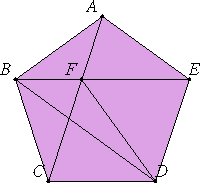# Proposition 7

If three angles of an equilateral pentagon, taken either in order or not in order, are equal, then the pentagon is equiangular.

First, let three angles A, B, and C taken in order in the equilateral pentagon ABCDE be equal to one another.

I say that the pentagon ABCDE is equiangular.Join AC, BE, and FD.

I.4

Now, since the two sides CB and BA equal the two sides BA and AE respectively, and the angle CBA equals the angle BAE, therefore the base AC equals the base BE, the triangle ABC equals the triangle ABE,and the remaining angles equal the remaining angles, namely those opposite the equal sides, that is, the angle BCA equals the angle BEA, and the angle ABE equals the angle CAB.

I.6

Hence the side AF also equals the side BF.

I.8

But the whole AC equals the whole BE, therefore the remainder FC equals the remainder FE. But CD also equals DE. Therefore the two sides FC and CD equal the two sides FE and ED, and the base FD is common to them, therefore the angle FCD equals the angle FED.

But the angle BCA was also proved equal to the angle AEB, therefore the whole angle BCD equals the whole angle AED. And, by hypothesis, the angle BCD equals the angles at A and B, therefore the angle AED also equals the angles at A and B. Similarly we can prove that the angle CDE also equals the angles at A, B, and C. Therefore the pentagon ABCDE is equiangular.

Next, let the given equal angles not be angles taken in order, but let the angles at the points A, C, and D be equal.

I say that in this case too the pentagon ABCDE is equiangular.

Join BD.

I.4

Then, since the two sides BA and AE equal the two sides BC and CD, and they contain equal angles, therefore the base BE equals the base BD, the triangle ABE equals the triangle BCD, and the remaining angles equal the remaining angles, namely those opposite the equal sides. Therefore the angle AEB equals the angle CDB.

I.5

But the angle BED also equals the angle BDE, since the side BE equals the side BD.

Therefore the whole angle AED equals the whole angle CDE.

But the angle CDE is, by hypothesis, equal to the angles at A and C, therefore the angle AED also equals the angles at A and C.

For the same reason the angle ABC also equals the angles at A, C, and D. Therefore the pentagon ABCDE is equiangular.

Therefore, if three angles of an equilateral pentagon, taken either in order or not in order, are equal, then the pentagon is equiangular.

Q.E.D.

## Guide

#### Use of this proposition

This proposition is needed in XIII.17 to show that the dodecahedron constructed there has equiangular pentagons as faces.